# Geometry Software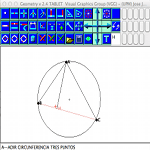Geometry is an educational tool for interactive geometry construction permits observation of geometric invariants dynamically modifying the position of the data.

Geometry is a program developed entirely in Java and distributed using a file “JAR” executable on any device that has a Java virtual machine. It has been tested on different operating systems and runtime environments, PDA-type mobile device including.## Program features

The different functions are accessible through a set of icons at the top.
The program can be found in two different states: Repeat command mode variational.Repeat Mode: Each time you press the left mouse button repeats the last command. It is useful to create a collection of points, to string lines that support these points, change the color of elements, create a set of circles …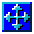Variational Mode. Allows you to run a single command or function, or drag and move the points of the figure to get variations of the same

The basic form of operation of the application is based on defining a set of points in which the geometry is supported. These points serve to further define other elements, as lines and circles.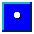Add points: To add a new point will click on this icon and then press in the screen in the position we want to initially address. Then we drag the element dynamicallyAdd segments: To define a line (segment) need to have two points. These may have been created with the function point, or result from the intersection of two elements. Will press the icon and then creating lines on screen select the two elements that define the ends, which can be points, lines (midpoint) the circles (the center), and existing intersections.Circumference by three points: The selected points will serve as a way point on the circumferenceDiameter circumference: The selected points will determine the diameter of the circleCircumference by center and radius: The first point is used as the center of the circle. The second will be a point on the circumference and the radius determined in the same

### Perpendicularity

Determine squareness features new auxiliary points used to determine angular lines with this condition.Perpendicular line from a point to another. First select the line to which must be perpendicular and then the point from which we draw the perpendicular.Perpendicular line at one end:

Parallelism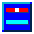Parallel line through a point: You can select a line or two crossing points to determine a direction. The last point selected will determine its positionParallel Dimension: This feature is not fully implemented

Intersection of elements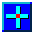Intersection of two lines: Allow to obtain the point of intersection of two lines or segments determined by its ends. Segments can exist or be determined by four points.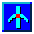Intersection between line and circle: Allows for up to two points of intersection between a line defined by the ends of a segment and a circumference. The order in which the data are defined determines the obtained solution.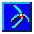Intersection of two circles: Lets get the two points of intersection of two circles. Again, the order in which data are selected determines which of the two solutions obtained.

### Display

The dot size can be changed, and the color of the elements. In the development version has been included modifying the line type.

### Deleting Items

The relationship between the geometric elements allow you to delete an item that is referenced by another. For this reason, only possible to delete elements in the reverse order in which they were generated, so that the geometric structure remains in a consistent state.